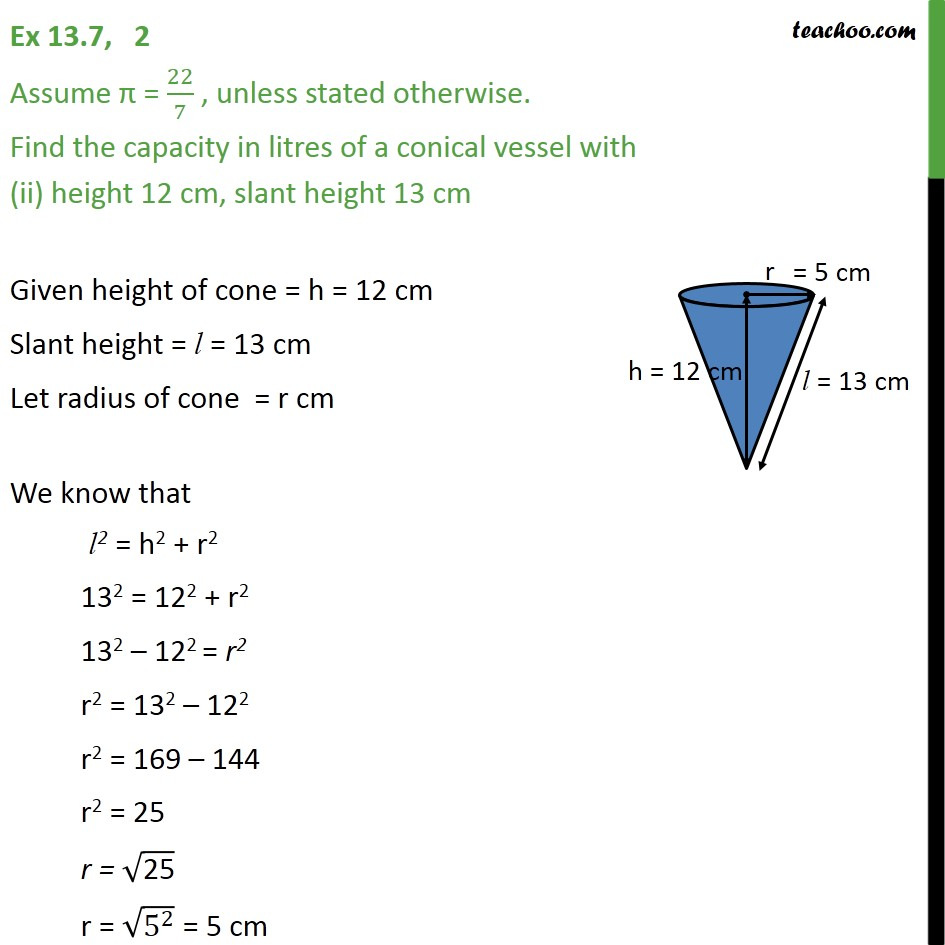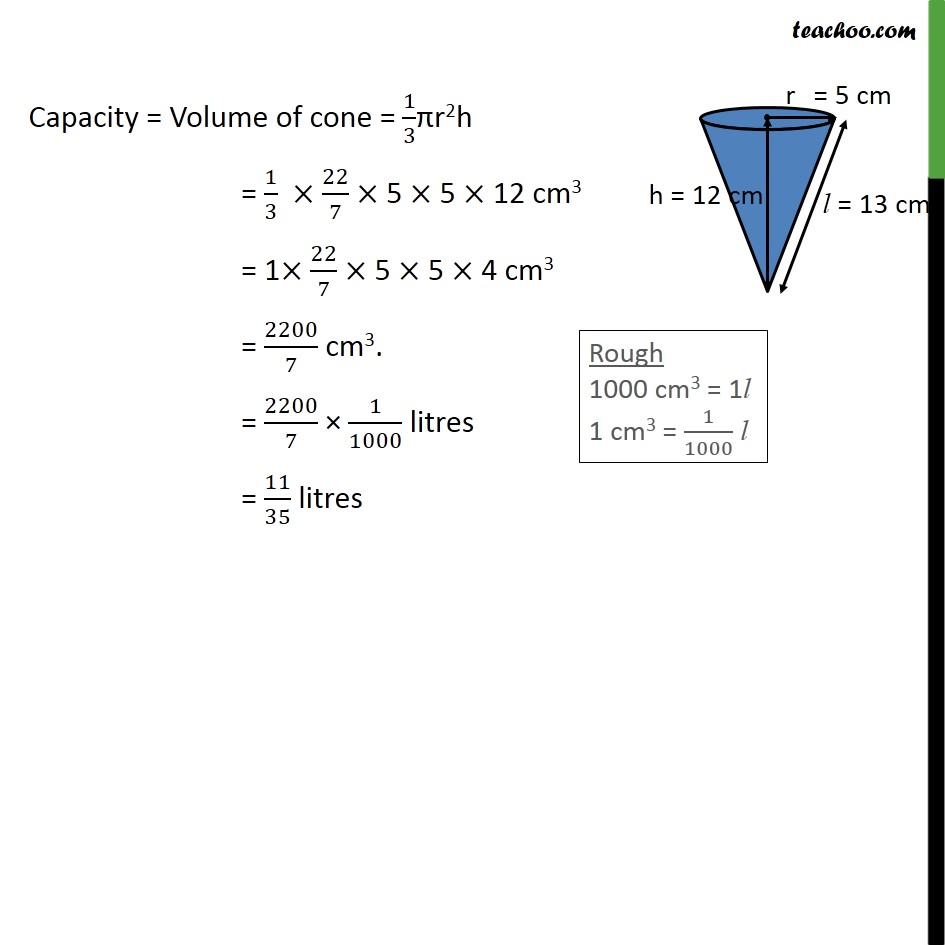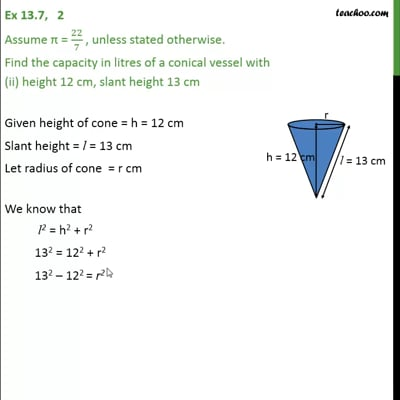Ex 11.3

Chapter 11 Class 9 Surface Areas and Volumes
Serial order wiseThis video is only available for Teachoo black users

Learn in your speed, with individual attention - Teachoo Maths 1-on-1 Class

### Transcript

Ex 11.3, 2 Assume π = 22/7 , unless stated otherwise. Find the capacity in litres of a conical vessel with (ii) height 12 cm, slant height 13 cm Given height of cone = h = 12 cm Slant height = l = 13 cm Let radius of cone = r cm We know that l2 = h2 + r2 132 = 122 + r2 132 – 122 = r2 r2 = 132 – 122 r2 = 169 – 144 r2 = 25 r = √("25" ) r = √(5^2 ) = 5 cm Capacity = Volume of cone = 1/3πr2h = 1/3 × 22/7 × 5 × 5 × 12 cm3 = 1× 22/7 × 5 × 5 × 4 cm3 = 2200/7 cm3. = 2200/7 × 1/1000 litres = 11/35 litres